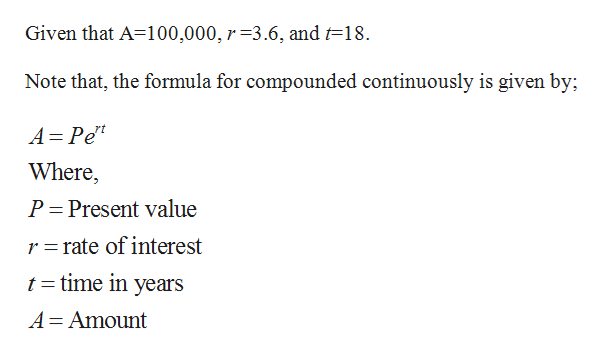# How much money, invested at an interest rate of r % per year compounded continuously, will amount to A dollars after t years? (Round your answer to the nearest cent.)A = 100,000,    r = 3.6,    t = 18

Question
1 views

How much money, invested at an interest rate of r % per year compounded continuously, will amount to A dollars after t years? (Round your answer to the nearest cent.)

A = 100,000,    r = 3.6,    t = 18
check_circle

Step 1help_outlineImage TranscriptioncloseGiven that A=100,000, r=3.6, and t=18. Note that, the formula for compounded continuously is given by; A= Pe" Where, P= Present value r = rate of interest t= time in years A= Amount fullscreen

### Want to see the full answer?

See Solution

#### Want to see this answer and more?

Solutions are written by subject experts who are available 24/7. Questions are typically answered within 1 hour.*

See Solution
*Response times may vary by subject and question.
Tagged in

### Other